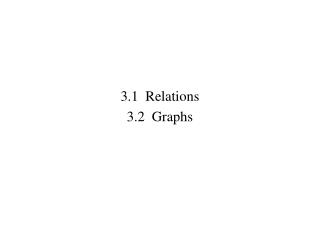# 3.1 Relations 3.2 Graphs - PowerPoint PPT PresentationDownload Presentation3.1 Relations 3.2 Graphs

3.1 Relations 3.2 GraphsDownload Presentation## 3.1 Relations 3.2 Graphs

- - - - - - - - - - - - - - - - - - - - - - - - - - - E N D - - - - - - - - - - - - - - - - - - - - - - - - - - -
##### Presentation Transcript

1. 3.1 Relations 3.2 Graphs

2. Objective: Find the Cartesian product of two sets.

3. Find the following Cartesian products.

4. Objective: List ordered pairs from a Cartesian product that satisfy a given relation. Any set of ordered pairs selected from a Cartesian product is a relation.

5. Objective: List the domain and the range of a relation.

6. List the domain and the range of the relation C {(a, 1), (b, 2), (c, 3), (e, 2)}. D {(2, 2), (1, 1), (1,2), (1, 3)}.

7. Objective: Use set-builder notation to define a relation.

8. Objective: Use set-builder notation to define a relation.

9. E Use the set {1, 2, 3, . . . , 10}.Find {x|5 < x < 7}. F Use the set Q X Q, where Q = {2, 3, 4, 5}.Find {(x, y)|x > 2 and y > 3}.

10. Objective: Graph ordered pairs of a relation Cartesian Coordinate System

11. Objective: Determine whether an ordered pair is a solution of an equation. Solution: An ordered pair such that when the numbers are substituted for the variables, a true equation is produced

12. Determine whether the given ordered pairs are solutions to the equation y = 3x - 1: G (7, 5) H (7, 20) I (0, 6)

13. Objective: Graph equations by plotting several solutions.

14. Graph the following relations

15. HW Quiz #3.1-2Thursday, October 9, 2014

16. Chapter 3Relations, Functions, and Graphs 3.3 Functions

17. Objective: Recognize functions and their graphs. A relation where each member of the domain is paired with exactly one member of the range is a function.

18. Objective: Recognize functions and their graphs.

19. Objective: Recognize functions and their graphs.

20. Objective: Recognize functions and their graphs. Function Not a Function

21. Objective: Use function notation to find the value of functions. FUNCTION MACHINE Pronounced “f of x”

22. Objective: Use function notation to find the value of functions. FUNCTION MACHINE

23. Objective: Find the domain of a function, given a formula for the function. When the function in R X R is given by a formula, the domain is understood to be all real numbers that are acceptable replacements. Finding the domain of a function  2 rules 1. Cannot let 0 be in the denominator 2. Cannot take a square root of a negative number

24. Objective: Find the domain of a function, given a formula for the function.

25. Find the domain of the following functions. State the domain using set-builder notation

26. HW #3.3-4Pg 120-121 1-27 odd, 30-36Pg 125-126 3-9 Odd, 11, 17, 21, 25, 27, 36-42

27. HW Quiz #3.3-4Thursday, October 9, 2014

28. Chapter 3Relations, Functions, and Graphs 3.4 Graphs of Linear Functions 3.5 Slope

29. Objective: Find the slope of a line containing a given pair of points. Slope is the measure of how steep a line is

30. Objective: Find the slope of a line containing a given pair of points. Slope is the measure of how steep a line is